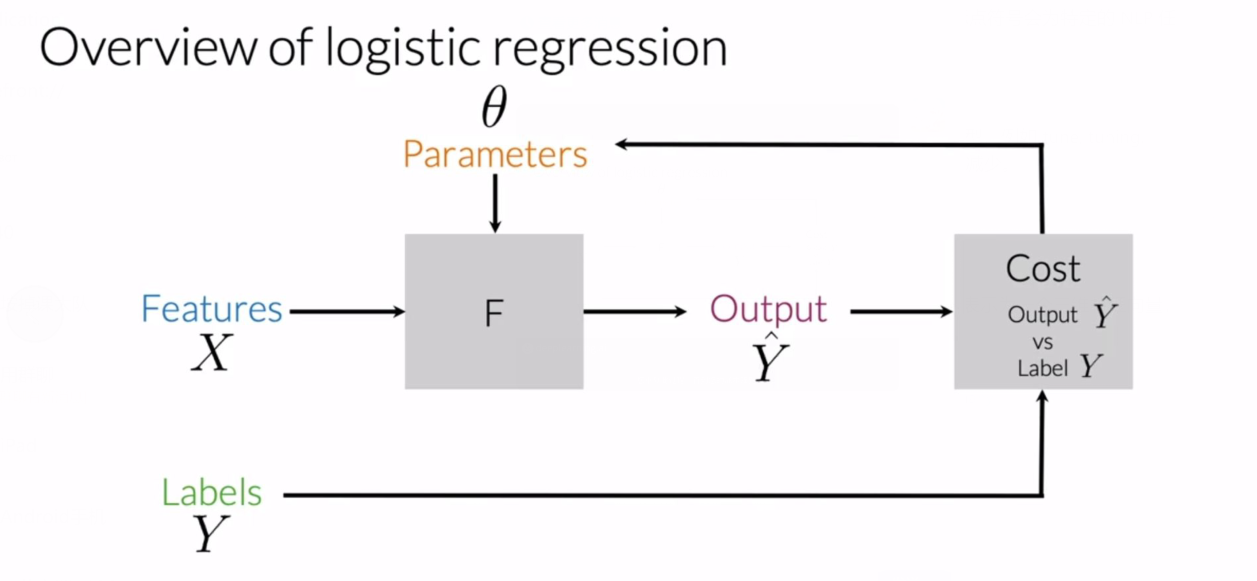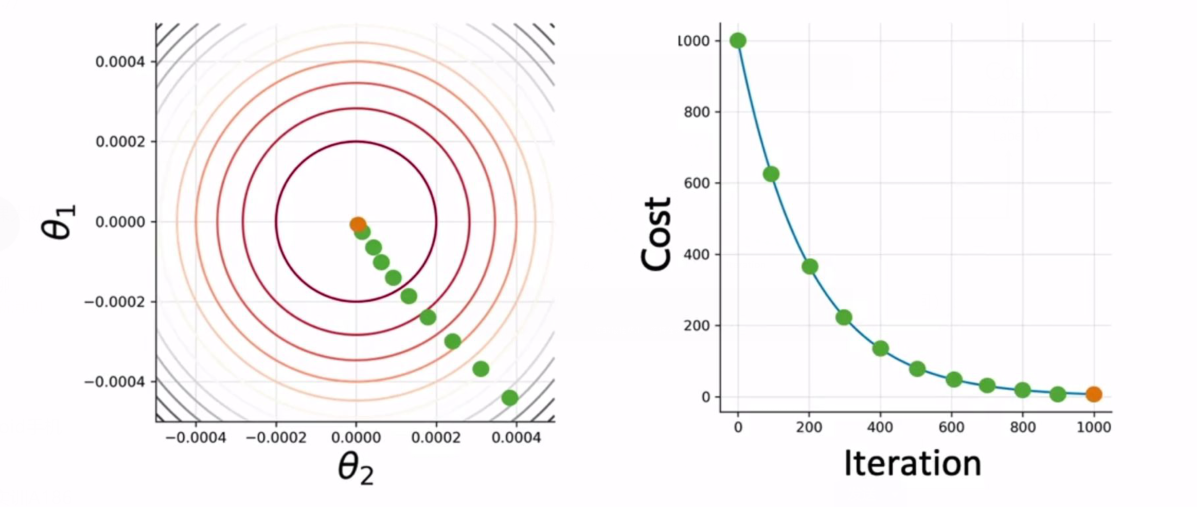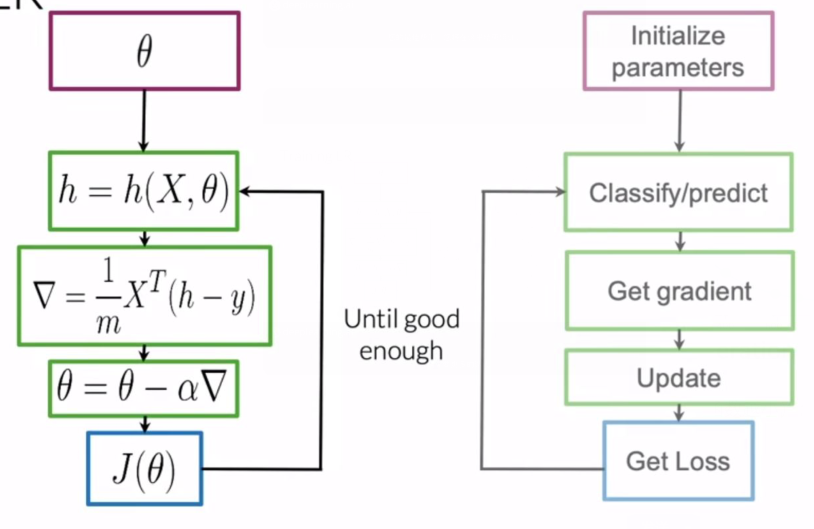# Sentiment analysis 情感分析

## Vocabulary 词汇表

$$V = {I, am, happy, because, learning, NLP, …, hated, the, movie}$$

## Feature extraction 特征提取

### 方法二：生成计数 Generate Count

$$freqs = (word, class) ⇒ frequency.$$

$$X_m = [1, \sum_w freqs(w,1), \sum_w freqs(w, 0)]$$

• 第一维元素 1 表示 偏置(bias)
• 第二维元素 $\sum_w freqs(w, 1)$ 表示词汇表中的每个词汇 $w$ 在正面情绪 (label=1) 的句子集合中出现的次数之和
• 第三维元素 $\sum_w freqs(w, 0)$ 表示词汇表中的每个词汇 $w$ 在负面情绪 (label=0) 的句子集合中出现的次数之和

## Generate Feature Matrix 生成特征矩阵

freqs = build_freqs(tweets, labels)    # build frequencies dictionary
X = np.zeros((m, 3))
for i in range(m):
p_tweet = process_tweet(tweet[i])
X[i,:] = extract_features(p_tweet, freqs)


## Logistic Regression 逻辑回归$$h(x^{(i)}, \theta) = \frac{1}{1 + e^{-\theta ^T x^{(i)}}}$$

## Training Logistic Regression Classifier 训练分类器• 首先取 $\theta_i$ 在任意一个点开始，得到 $h = h(X, \theta)$
• 然后计算 $\theta_1, \theta_2$ 在该点的梯度 $\nabla = \frac{1}{m} X^T (h-y)$
• 使用梯度更新参数 $\theta = \theta-\nabla$
• 然后计算 loss （损失）函数 $J(\theta)$，重复上述步骤直到 $J(\theta)$ 足够好。## Testing Logistic Regression 测试逻辑回归

• 首先计算参数为 $\theta$、自变量为 $X_{val}$ 的 $sigmoid$ 函数
• 计算 $pred = (h(X_{val}, \theta) \ge 0.5)$，0.5 为阈值
• 对比 $pred$ 和 $Y{val}$ ，计算准确率 (accuracy) = $\sum{i=1}^{|val|} \frac{(pred^i == y_{val}^{i})}{m}$

## Cost Function 代价函数

$$J(\theta) = -\frac{1}{m} \sum_{i=1}^m [y^{(i)} \log h(x^{(i)}, \theta) + (1 - y^{(i)}) \log (1 - h(x^{(i)}, \theta))]$$

$y$ 的取值可能是 0 或 1，考虑第一项对代价函数的贡献：

• 如果 $y^{(i)}$ 为 0，那么第一项无论 $h$ 取何值都为 0；
• 如果 $y^{(i)}$ 为 1，且 $h$ 接近于 1，则第一项会趋近于 0；
• 如果 $y^{(i)}$ 为 1，且 $h$ 接近于 0，则第一项中的对数会趋于 $-\infty$，从而使得第一项趋于 $-\infty$.

• 如果 $y^{(i)}$ 为 1，则 $1-y^{(i)}$ 为 0，那么第二项无论 $h$ 取何值都为 0；
• 如果 $y^{(i)}$ 为 0，且 $h$ 接近于 0，则第二项会趋近于 0；
• 如果 $y^{(i)}$ 为 0，且 $h$ 接近于 1，则第二项中的对数会趋于 $-\infty$﻿，从而使得第二项趋于 $-\infty$﻿.